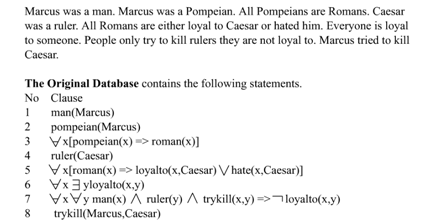Pages

Resolution

Resolution yields a complete inference algorithm when coupled with any complete search algorithm.Resolution makes use of the inference rules. Resolution performs deductive inference.Resolution uses proof by contradiction. One can perform Resolution from a Knowledge Base. A Knowledge Base is a collection of facts or one can even call it a database with all facts.

Resolution Algorithm:

Resolution basically works by using the principle of proof by contradiction. To find the conclusion we should negate the conclusion. Then the resolution rule is applied to the resulting clauses. Each clause that contains complementary literals is resolved to produce a two new clause,which can be added to the set of facts (if it is not already present).This process continues until one of the two things happen:
·         There are no new clauses that can be added
·         An application of the resolution rule derives the empty clause

An empty clause shows that the negation of the conclusion is a complete contradiction ,hence the negation of the conclusion is invalid or false or the assertion is completely valid or true.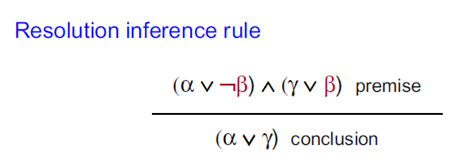Steps for Resolution Refutation

1    1. Convert all the propositions of KB to clause form (S).
2. Negate a and convert it to clause form. Add it to S.
3. Repeat until either a contradiction is found or no progress can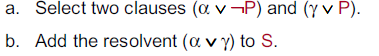Unification:

In propositional logic it is easy to determine that two literals can not both be true at the same time. Simply look for L and ~L . In predicate logic, this matching process is more complicated, since bindings of variables must be considered.

For example man (john) and man(john) is a contradiction while man (john) and man(Himalayas) is not. Thus in order to determine contradictions we need a matching procedure that compares two literals and discovers whether there exist a set of substitutions that makes them identical . There is a recursive procedure that does this matching . It is called Unification algorithm.

In Unification algorithm each literal is represented as a list, where first element is the name of a predicate and the remaining elements are arguments. The argument may be a single element (atom) or may be another list. For example we can have literals as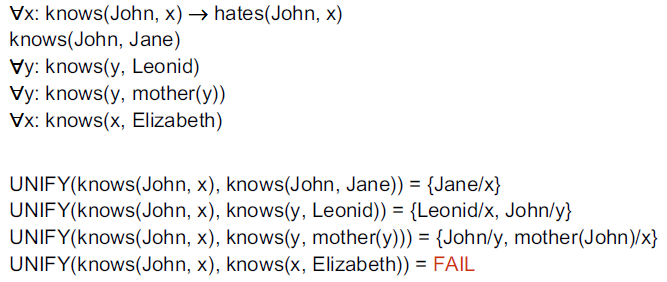The Unification algorithm is listed below as a procedure UNIFY (L1, L2). It returns a list representing the composition of the substitutions that were performed during the match. An empty list NIL indicates that a match was found without any substitutions. If the list contains a single value F, it indicates that the unification procedure failed.

UNIFY (L1, L2)

1. if L1 or L2 is an atom part of same thing do

(a) if L1 or L2 are identical then return NIL

(b) else if L1 is a variable then do

(i) if L1 occurs in L2 then return F else return (L2/L1)

else if L2 is a variable then do

(i) if L2 occurs in L1 then return F else return (L1/L2)

else return F.

2. If length (L!) is not equal to length (L2) then return F.

3. Set SUBST to NIL

( at the end of this procedure , SUBST will contain all the substitutions used to unify L1 and L2).

4. For I = 1 to number of elements in L1 do

i) call UNIFY with the i th element of L1 and I’th element of L2, putting the result in S

ii) if S = F then return F

iii) if S is not equal to NIL then do

(A) apply S to the remainder of both L1 and L2

(B) SUBST := APPEND (S, SUBST) return SUBST.

Resolution in Prepositional Logic

We start by converting this first sentence into conjunctive normal fom. We don’t actually have to do anything. It’s already in the right form. Now, “P implies R” turns into “not P or R”. Similarly, “Q implies R” turns into “not Q or R .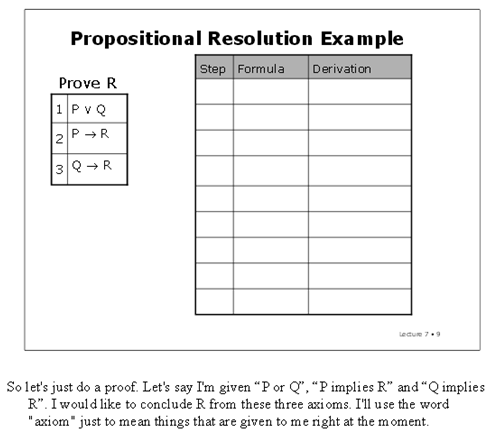We start by converting this first sentence into conjunctive normal fom. We don’t actually have to do anything. It’s already in the right form. Now, “P implies R” turns into “not P or R”. Similarly, “Q implies R” turns into “not Q or R .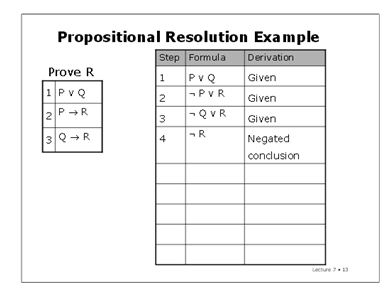Now we want to add one more thing to our list of given statements. What's it going to be? Not R. Right?We're gong to assert the negation of the thing we're trying to prove. We'd like to prove that R follows from these things. But what we're going to do instead is say not R, and now we're trying to prove false. And if we manage to prove false, then we will have a proof that R is entailed by the assumptions.
And now, we look for opportunities to apply the resolution rule. You can do it in any order you like (though some orders of application will result in much shorter proofs than others). We can apply resolution to lines 1 and 2, and get “Q or R” by resolving away P. And we can take lines 2 and 4, resolve away R, and get “not P.” Similarly, we can take lines 3 and 4, resolve away R, and get “not Q”. By resolving away Q in lines 5 and 7, we get R. And finally, resolving away R in lines 4 and 8, we get the empty clause, which is false. We’ll often draw this little black box to indicate that we’ve reached the desired contradiction.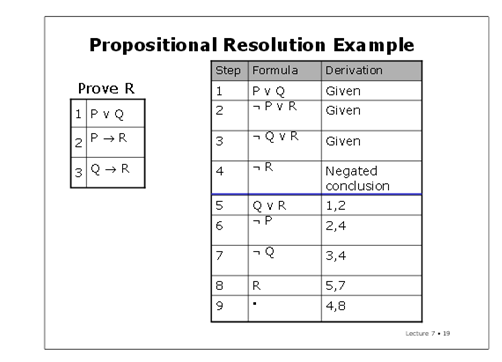Resolution in Predicate Logic

Consider the example,

“All Romans who know Marcus either hate Caesar or think that anyone who hates anyone is crazy”

Step 1: Convert the given statements in Predicate/Propositional Logic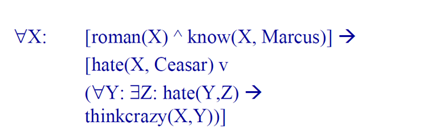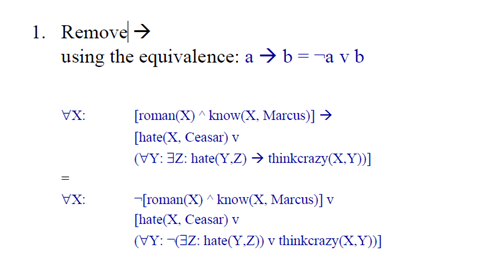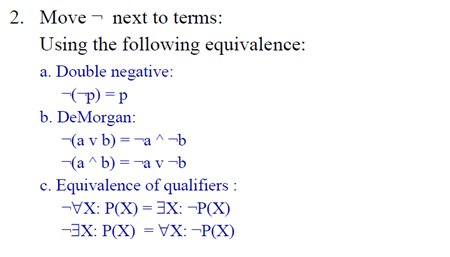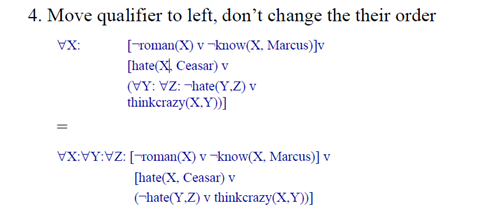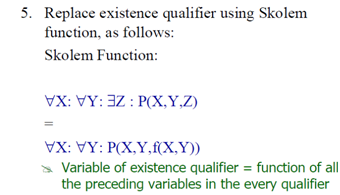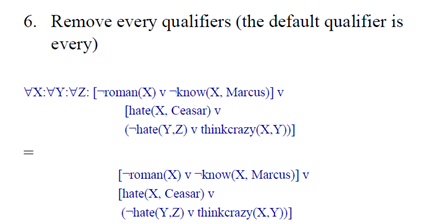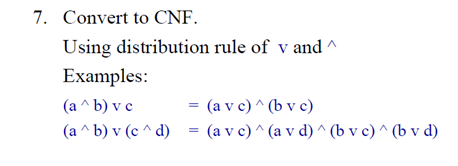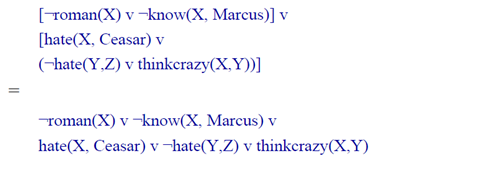Consider the following example,

Example1: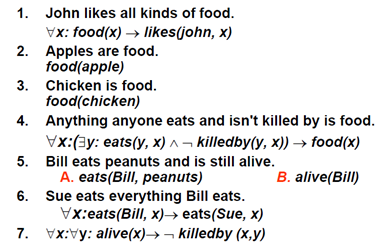Clause Form: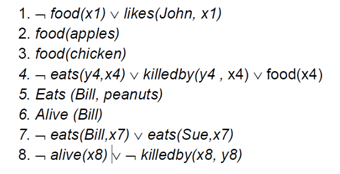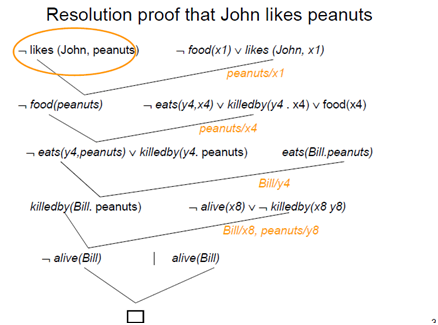Example 2: